Author: Oscar Cronquist Article last updated on May 06, 2022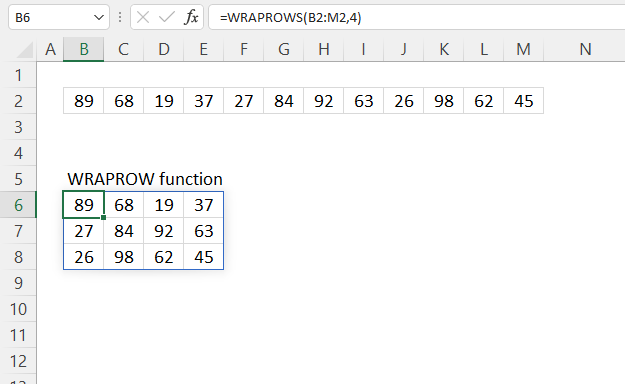The WRAPROWS function rearranges values from a single row to a 2D cell range.

Dynamic array formula in cell B8:

=WRAPROWS(B2:M2, 4)

The WRAPROWS function is available to Excel 365 users and is in the "Array manipulation" category.

## 2. WRAPROWS Function Arguments

 vector Required. The source cell range or array. wrap_count Required. The number of values per row, the last row may be smaller. [pad_with] Optional. Value to pad with if there is an insufficient number of values in the last row. The default value is #N/A!

## 3. WRAPROWS Function example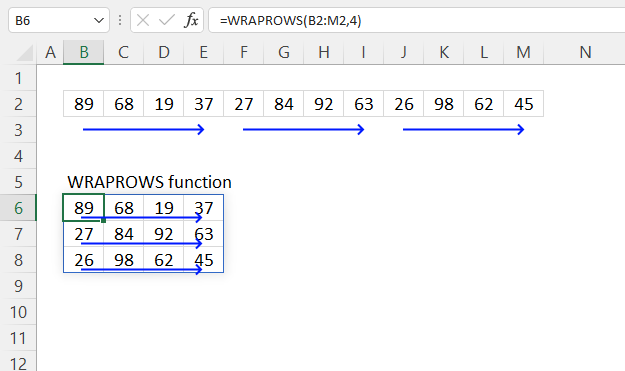The picture above shows how the WRAPROWS function rearranges values to fit the requirement specified in the second argument: wrap_count.

Dynamic array formula in cell B8:

=WRAPROWS(B2:M2, 4)
Note! If any of the values in the source range change, the values in the WRAPROWS function output change as well.

### 3.1 Explaining formula

vector - B2:E4
wrap_count - 4

#### Step 3 - Evaluate function

WRAPROWS(B2:M2, 4)

becomes

WRAPROWS({89, 68, 19, 37, 27, 84, 92, 63, 26, 98, 62, 45})

and returns

{89, 68, 19, 37; 27, 84, 92, 63; 26, 98, 62, 45}.

## 4. WRAPROWS Function example - pad with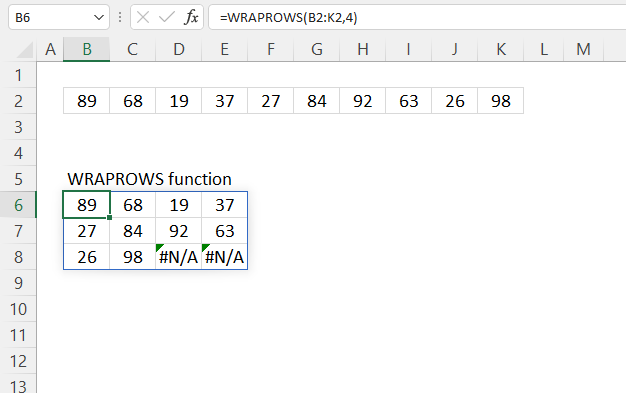#N/A errors show up in empty cells on the last row, use the [pad_with] argument to populate empty cells.

Formula in cell B6:

=WRAPROWS(B2:K2, 4)

The image below demonstrates how to populate empty cells with the text string "A".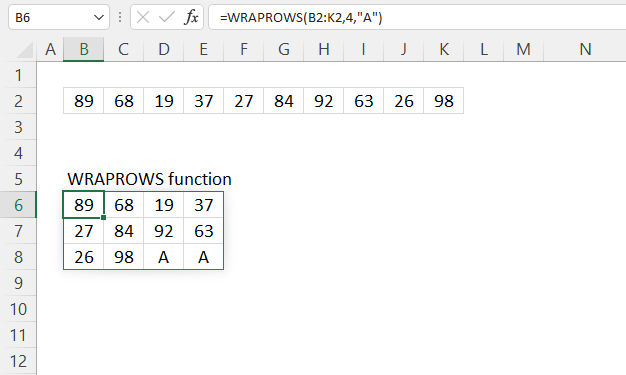Formula in cell B6:

=WRAPROWS(B2:K2, 4, "A")

## 5. WRAPROWS function alternative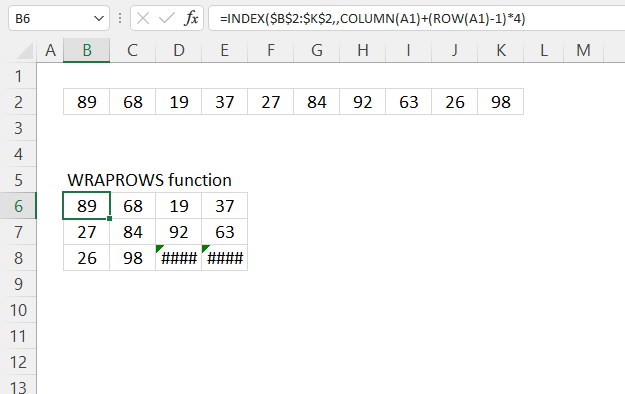This formula wraps the values in cell range B2:K2 to fit four columns. Change the bolded number in the formula below to adjust the number of columns.

Formula in cell B6:

=INDEX(\$B\$2:\$K\$2,,COLUMN(A1)+(ROW(A1)-1)*4)

### 5.1 Explaining formula in cell B6

#### Step 1 - Calculate the number of columns in a given cell range

The COLUMN function returns the column number of the top-left cell of a cell reference.

COLUMN(reference)

COLUMN(A1) returns 1.

#### Step 2 - Calculate column number of relative cell reference

The ROW function returns the row number of a cell reference.

ROW(reference)

ROW(A1)-1

becomes

1 - 1 equals 0 (zero).

#### Step 3 - Multiply

The asterisk lets you multiply numbers in an Excel formula.

The parentheses controls the order of calculation, we want to subtract before we multiply.

(ROW(A1)-1)*4

becomes

0*4 equals 0 (zero)

#### Step 4 - Calculate column number

The INDEX function returns a value from a cell range, you specify which value based on a row and column number.

INDEX(array[row_num][column_num], [area_num])

INDEX(\$B\$2:\$K\$2,,COLUMN(A1)+(ROW(A1)-1)*4)

becomes

INDEX(\$B\$2:\$K\$2,,1)

and returns 89 in cell B6.

## 6. WRAPROWS function - values in random order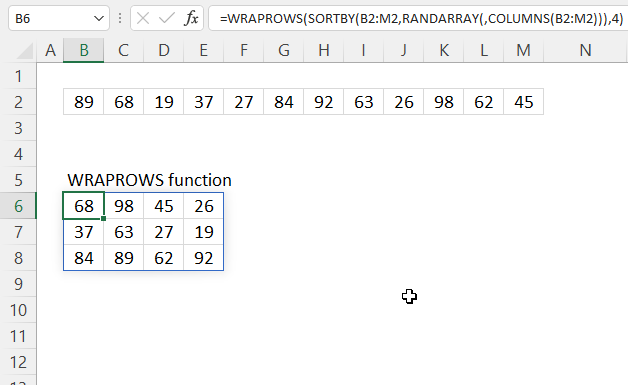The image above shows a formula that rearranges values in random order with four columns.

Dynamic array formula in cell B6:

=WRAPROWS(SORTBY(B2:M2, RANDARRAY(, COLUMNS(B2:M2))), 4)

### 6.1 Explaining formula in cell B8

#### Step 1 - Calculate the number of cells in cell ref

The COLUMNS function returns the number of columns in a given cell range.

COLUMNS(array)

COLUMNS(B2:M2)

returns 12.

#### Step 2 - Create random decimal values

The RANDARRAY function returns a table of random numbers across rows and columns.

RANDARRAY([rows], [columns], [min], [max], [whole_number])

RANDARRAY(, COLUMNS(B2:M2))

becomes

RANDARRAY(, 12)

and returns

{0.732441255476154, 0.953587479053817, ... , 0.0191675082920368}

#### Step 3 - Sort values based on random decimals

The SORTBY function allows you to sort values from a cell range or array based on a corresponding cell range or array.

SORTBY(array, by_array1, [sort_order1], [by_array2, sort_order2],…)

SORTBY(B2:M2, RANDARRAY(, COLUMNS(B2:M2)))

becomes

SORTBY(B2:M2, {0.732441255476154, 0.953587479053817, ... , 0.0191675082920368})

and returns

#### Step 4 - Create random values

The RANDARRAY function returns a table of random numbers across rows and columns.

RANDARRAY([rows], [columns], [min], [max], [whole_number])

RANDARRAY(, ROWS(B2:E4)*COLUMNS(B2:E4))

becomes

RANDARRAY(, 12)

and returns 12 random decimal values:

{0.464680665246457, 0.768212043461448, ... ,0.281771048521311}

#### Step 6 - Sort values in random order

The SORTBY function allows you to sort values from a cell range or array based on a corresponding cell range or array.

SORTBY(array, by_array1, [sort_order1], [by_array2, sort_order2],…)

SORTBY(B2:M2, RANDARRAY(, COLUMNS(B2:M2)))

becomes

SORTBY(B2:M2, {0.732441255476154, 0.953587479053817, ... , 0.0191675082920368})

becomes

SORTBY({89, 68, 19, 37, 27, 84, 92, 63, 26, 98, 62, 45}, {0.732441255476154, 0.953587479053817, ... , 0.0191675082920368})

{63, 45, 27, 68, 84, 26, 92, 62, 98, 37, 89, 19}

#### Step 5 - Wrap values

WRAPROWS(SORTBY(B2:M2, RANDARRAY(, COLUMNS(B2:M2))), 4)

becomes

WRAPROWS({63, 45, 27, 68, 84, 26, 92, 62, 98, 37, 89, 19}, 4)

and returns

{63,45,27,68;
84,26,92,62;
98,37,89,19}

Press F9 to recalculate values, this creates a new random order.

## 7. WRAPROWS Function - multiple source ranges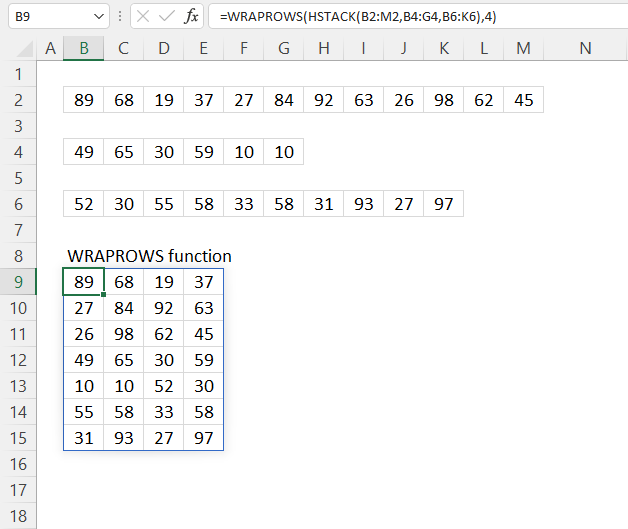The graphic above demonstrates a formula that merges values from cell ranges B2:M2, B4:G4, and B6:K6, then wraps the values to a 2D range with max four values wide.

Dynamic array formula in cell B6:

=WRAPROWS(HSTACK(B2:M2, B4:G4, B6:K6), 4)

### 8.1 Explaining formula

#### Step 1 - Stack values horizontally

The HSTACK function lets you combine cell ranges or arrays, it joins data to the first blank cell to the right of a cell range or array (horizontal stacking)

HSTACK(array1, [array2],...)

HSTACK(B2:M2, B4:G4, B6:K6)

becomes

HSTACK({89, 68, 19, 37, 27, 84, 92, 63, 26, 98, 62, 45}, {49, 65, 30, 59, 10, 10}, {52, 30, 55, 58, 33, 58, 31, 93, 27, 97})

and returns

{89, 68, 19, 37, 27, 84, 92, 63, 26, 98, 62, 45, 49, 65, 30, 59, 10, 10, 52, 30, 55, 58, 33, 58, 31, 93, 27, 97}.

#### Step 2 - Wrap values to rows

WRAPROWS(HSTACK(B2:M2, B4:G4, B6:K6), 4)

becomes

WRAPROWS({89, 68, 19, 37, 27, 84, 92, 63, 26, 98, 62, 45, 49, 65, 30, 59, 10, 10, 52, 30, 55, 58, 33, 58, 31, 93, 27, 97}, 4)

and returns

{89, 68, 19, 37;
27, 84, 92, 63;
26, 98, 62, 45;
49, 65, 30, 59;
10, 10, 52, 30;
55, 58, 33, 58;
31, 93, 27, 97}